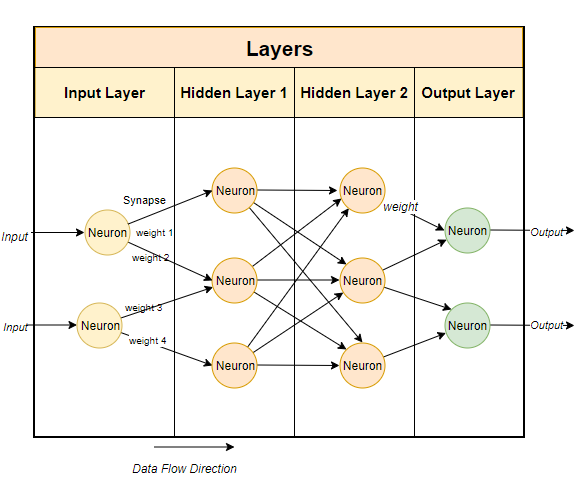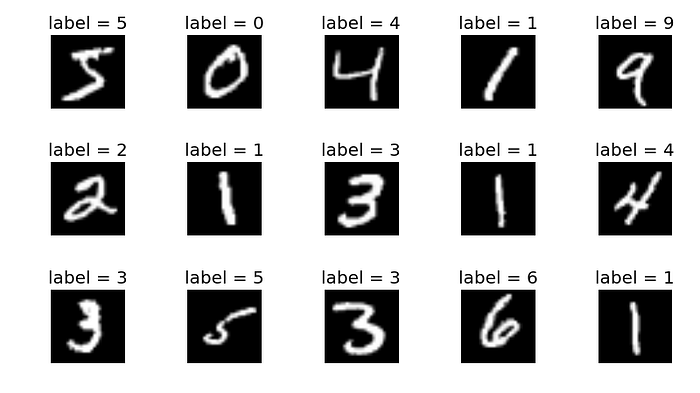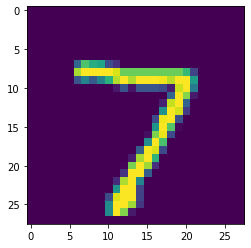# Implementing Convolutional Neural Networks with Keras# Implementing Convolutional Neural Networks with Keras

## Intro

Keras is a Deep Learning library for Python, after learning Intro to Neural Networks and Intro to Convolutional Neural Networks from Victor Zhou, These two posts produce implementations of Neural Networks and CNN from scratch (using only numpy), To refresh the knowledge while reviewing it, I decided to write a Note.

A Neural Network consists a bunch of Neurons connected together with differentiate by Layers, i.e. `Input Layers`, `Hidden Layers(Conv, Pooling, Activation Functions Layers, Fully Connected Layer)` and `Output Layers`. Hidden layers make neural networks superior to most of the machine learning algorithms.Training a Neural Network model, in a nutshell, is changing the network’s `weights` and `biases` to decrease the loss of the model, actually, the goals of every types of Neural Networks is that. We use loss functions, i.e. `MSE loss function (Mean Squared Error)`, `Cross-entropy cost function` to calculate loss, now we have loss, with the help of backpropagation (反向传播) algorithm and gradient descent (梯度下降) algorithm (opt-algorithm called stochastic gradient descent) to alternate parameters. This kind of Neural Network is called Feedforward Neural Network (前馈神经网络).

EN 中文术语对照 Keras Loss Function
Mean Squared Error 均方误差 `mse`
Binary Cross-entropy 二维交叉熵 `binary_crossentropy`
Categorical Cross-entropy 多分类交叉熵 `categorical_crossentropy`

After iterations of many times, our Neural Network increase the accuracy step by step. Finally we save the trained model. That’s it — a brief process of Training a Neural Network.

## Implements

If you’d like to learn it in detailed, reading this article is a good choice Intro to Neural Networks, you need to learn Partial Derivative if you want to calculate together with this article. But for now, I would like to use `tensorflow.Keras` to implement it without caring about how it works inside.

What we will do is to classify handwritting digits(0-9) into labels, we introduce the dataset from `mnist` , it looks like this.Now that we can see we want to build a classifier, actually that is a `model`. Let’s start implementing it as following.

At first, we need to import packages we need, you may use `pip install <package-name>` to install it if you do not have. `mnist` is a dataset which contains 60000 handwriting Arabic numbers from 0 to 9 with labels for testing, and we import `keras` , from `keras` we need to import `models.Sequential` to create `Sequential` model, since our CNN will be a linear stack of layers, `models.Dense` to create full-connected layers, we also need `utils.to_categorical` to get the prediction metric. Moreover, to import `matplotlib.pyplot`, we can take a glance at `mnist` dataset.

``````import numpy as np
import mnist

from tensorflow import keras
from tensorflow.keras.models import Sequential
from tensorflow.keras.layers import Dense
from tensorflow.keras.utils import to_categorical

import matplotlib.pyplot as plt
``````

### Build Model

Then it’s time to build a model, we need to use `Sequential` class. Designing `model_cnn` , I chose a sequence of `Conv -> MaxPool -> Conv -> Softmax` , what we need to mention is that only declare `input_shape` once in the first layer is OK. As this article will not expand in explaining the details about every Layers, you can check this article from Keras Layers API if you want to learn about it. Breifly Speaking, `Conv` is for reserving characteristics of data, `Pool` is for increasing receptive field (感受野) or make the data more unified, it can shrink the resolution. `Softmax` is for outputing the Layers before in `10` possiblilities which represent each digits(0-9).

• `num_filters`, `filter_size`, and `pool_size` are self-explanatory variables that set the hyperparameters for our CNN.
• The output Softmax layer has 10 nodes, one for each class.
``````num_filters = 8
filter_size = 3
pool_size = 2

model_cnn = Sequential([
Conv2D(num_filters, filter_size, input_shape=(28, 28, 1)),
MaxPooling2D(pool_size=pool_size),
Conv2D(num_filters, filter_size),
MaxPooling2D(pool_size=pool_size),
Flatten(),
Dense(10, activation='softmax'),
])
``````

### Compile Model

At the meanwhile, we need to add some parameters to configure the training process.Our `model_cnn` including optimizer, loss function and a list of metrics which contains accuracy since this is a classification problem.

``````model_cnn.compile(
loss='categorical_crossentropy',
metrics=['accuracy'],
)
``````

### Train Model

OK, It’s time to train it, getting data then centralize (or normalize), We’ll also reshape each image from `(28, 28)` to `(28, 28, 1)` because Keras requires the third dimension. then setting epochs, i.e. training times.

``````# Import images.
train_images = mnist.train_images()
train_labels = mnist.train_labels()
test_images = mnist.test_images()
test_labels = mnist.test_labels()

# Normalize the images.
train_images = (train_images / 255) - 0.5
test_images = (test_images / 255) - 0.5

# Reshape
train_images = np.expand_dims(train_images, axis=3)
test_images = np.expand_dims(test_images, axis=3)

model_cnn.fit(
train_images,										# x_train
to_categorical(train_labels),   # y_train
epochs=3,
validation_data=(test_images, to_categorical(test_labels))
)

# Save the model
model_cnn.save_weights('model_cnn.h5')
``````

You can take a glance at `test_images`

``````plt.imshow(test_images)
``````Now, `Shift + Enter` to run it. If you were not go wrong, output will be like that.

``````Epoch 1/3
1875/1875 [==============================] - 9s 5ms/step - loss: 0.2870 - accuracy: 0.9160 - val_loss: 0.1344 - val_accuracy: 0.9585
Epoch 2/3
1875/1875 [==============================] - 9s 5ms/step - loss: 0.1276 - accuracy: 0.9613 - val_loss: 0.0878 - val_accuracy: 0.9724
Epoch 3/3
1875/1875 [==============================] - 8s 4ms/step - loss: 0.0990 - accuracy: 0.9697 - val_loss: 0.0794 - val_accuracy: 0.9750
``````

### Using Model

Using the trained model to make predictions is easy: we pass an array of inputs to `predict()` and it returns an array of outputs. `argmax()` is a method turns `[.1, .1, .15, .12, .05, .08, .09, .11, .09, .11]` to `2` (max index)

``````# Predict on the first 5 test images.
predictions = model_cnn.predict(test_images[:5])

# Print our model's predictions.
print(np.argmax(predictions, axis=1)) # [7, 2, 1, 0, 4]

# Check our predictions against the ground truths.
print(test_labels[:5]) # [7, 2, 1, 0, 4]
``````

## Conclusion

Steps are `Build Model -> Compile Model -> Train Model -> Predict`, to make a more accurate result, designers should add some Layers in specific orders, or use another kind of layers like `activation function`

Meanwhile, changing Network Depth, Dropout, Full-connected layers and convolution parameters can also make a better result.

### Project Full Code

``````import numpy as np
import mnist

from tensorflow import keras
from tensorflow.keras.models import Sequential
from tensorflow.keras.layers import Dense
from tensorflow.keras.utils import to_categorical

import matplotlib.pyplot as plt

num_filters = 8
filter_size = 3
pool_size = 2

model_cnn = Sequential([
Conv2D(num_filters, filter_size, input_shape=(28, 28, 1)),
MaxPooling2D(pool_size=pool_size),
Conv2D(num_filters, filter_size),
MaxPooling2D(pool_size=pool_size),
Flatten(),
Dense(10, activation='softmax'),
])

model_cnn.compile(
loss='categorical_crossentropy',
metrics=['accuracy'],
)

# Import images.
train_images = mnist.train_images()
train_labels = mnist.train_labels()
test_images = mnist.test_images()
test_labels = mnist.test_labels()

# Show images.
plt.imshow(test_images)

# Normalize the images.
train_images = (train_images / 255) - 0.5
test_images = (test_images / 255) - 0.5

# Reshape
train_images = np.expand_dims(train_images, axis=3)
test_images = np.expand_dims(test_images, axis=3)

model_cnn.fit(
train_images,										# x_train
to_categorical(train_labels),   # y_train
epochs=3,
validation_data=(test_images, to_categorical(test_labels))
)

# Save the model
model_cnn.save_weights('model_cnn.h5')
``````